# The Universe of Discourse

Thu, 06 Apr 2006

Pick's theorem
In a recent article, I discussed Hero's formula for the area of a triangle in terms of its sides, and I said it was an oddity that didn't seem like any other formula in geometry. Pick's theorem is another such oddity, although not at all like Hero's.

Pick's theorem concerns the area of so-called "lattice polygons". These are simply polygons whose vertices all lie at points whose coordinates are integers. Such points are called "lattice points". Here it is:

Let P be a lattice polygon. Let b be the number of lattice points that lie on the edges of the polygon, and i be the number of lattice points inside the polygon. Then the area of the polygon is exactly b/2 + i - 1.

I think this should be at least a bit surprising. It implies that every lattice polygon has an area that is an integer multiple of ½, which I would not have thought was obvious.

Some examples now. Pick's theorem is obviously true for rectangles: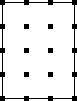In the example above, b = 14 and i = 6, so Pick's theorem says that the area is 14/2 + 6 - 1 = 12, which is correct. In general, an m×n rectangle has (m-1)(n-1) lattice points inside, and 2m + 2n on the edges, so by Pick's theorem its area is (2m + 2n)/2 + (mn - m - n + 1) - 1 = mn.

We can cut the rectangle in half, and it still works: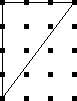b is now 8 and i is 3, so Pick's theorem predicts an area of 8/2 + 3 - 1 = 6, which is still correct. This works even when the diagonal cuts through some lattice points: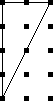Here b is still 8, but i is only 1, so Pick predicts an area of 8/2 + 1 - 1 = 4.

It works for figures without right angles: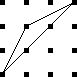Here b=5 and i=0, for a total area of 3/2. We can check the area manually as follows: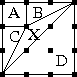The entire square has area 9. Regions A, B, and C each have area 1, and D has area 4½. That leaves X = 1½ as Pick's theorem says.

It works for more complicated figures too. Here we have b=7 and i=5 for a total area of 7½: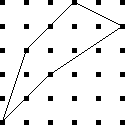It works for non-convex polygons: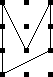It does not, however, work for polygons with holes. The figure below is the same as the first triangle in this article (which had an area of of 6) except that it has a hole of size ½ chopped out of it. It also has three fewer interior points and three more boundary points than the original triangle, for a total net loss of 3 - 3/2 = 3/2.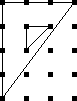To fix Pick's theorem for non-simply-connected polygons, you need to say that each hole adds an extra -1 to the total area.

The proof of Pick's theorem isn't hard. You start by proving it that it holds for all triangles that have two sides parallel to the x and y axes. Then you prove it for all triangles, using a subtraction argument like the one I used above for triangle X. Finally, you use induction to prove it for more complicated regions, which can be built up from triangles.

But the funny thing about Pick's theorem is that you can guess it even without a proof. Suppose someone told you that there was a formula for the size of a polygon in terms of the number of lattice points on the boundary and in the interior. Well, each interior point is surrounded by a square of area 1, which is typically inside the polygon: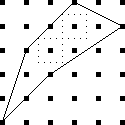So each such point should contribute about 1 to the area. Of course, not all do; some will contribute a little less: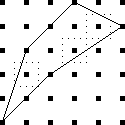But there will also be parts of the polygon that are not near any interior points: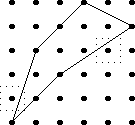The points outside the polygon whose squares are partly inside (which count less than they should) will tend to balance out the contributions of the points inside whose squares are partly outside (which count more than they should.)

The squares around a point on the edge (but not the vertex) of the polygon will always be half inside, half outside, so that such a point will contribute exactly half of a square to the total: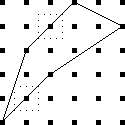The vertices are a little funny. They also contribute about ½, for the same reason that the edge points do. But convex vertices contribute rather less than ½: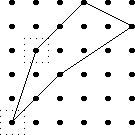While concave vertices contribute a bit more: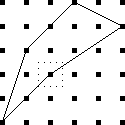So we need to adjust the contribution of the vertices away from the ideal of ½ each. The adjustment is positive when angle is more than 180°, in which case the path at the vertex turns clockwise, and negative when the angle is less than 180°, when the path turns counterclockwise. But any polygon makes exactly one complete counterclockwise turn, so the total adjustment is exactly one square's-worth, or -1, and this is where the fudge factor of -1 comes from. (I've known about Pick's theorem for twenty years, but I never knew where the -1 came from until just now.) Viewed in this light, it makes perfect sense that a hole in the polygon should subtract an extra unit of space, since it's adding an extra complete turn.

I made the diagrams for this article with a picture-drawing tool, originally designed at Bell Labs, called pic. The source code files for the illustrations were all named things like pick.pic, and the command to compile them to PostScript was pic pick.pic. This was really confusing.